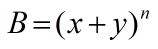Request a Tool

Binomial Theorem Calculator

Binomial Theorem Calculator use to calculate binomial theorem from x, y, and n values.

Binomial Theorem
0

Formula• B = Binomial theorem
• x = Value of x
• y = Value of y
• n = It is the Power

Defination / Uses

The binomial theorem states that the nth power of the sum of two numbers a and b may be written as the sum of n + 1 terms of the form for every positive integer n.

The word binomial expression refers to a polynomial with two terms in mathematics. These two terms are always separated by a plus or a minus, and they appear in a series. A binomial theorem is the name for this series. It's also known as a binomial theorem formula, which allows a polynomial with two terms to be expanded. This theorem also describes how to extend an expression that has been raised to an infinite power. This type of extension can be done with a binomial theorem calculator. The binomial theorem states that the nth power of the totality of two integers a and b may be articulated as the sum of n + 1n+1 relations of the form for every positive number n.